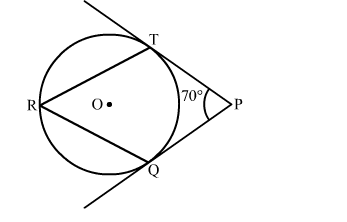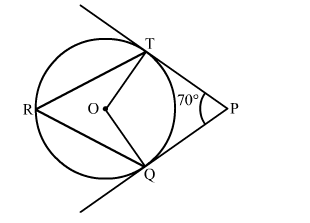# In the given figure, O is the centre of a circle PT and PQ are tangents to the circle from an external point P.`
Question:

In the given figure, O is the centre of a circle PT and PQ are tangents to the circle from an external point P. If ∠TPQ = 70 then ∠TRQSolution:

Construction: Join OQ and OTWe know that the radius and tangent are perperpendular at their point of contact
∵∠OTP = ∠OQP = 90
$\therefore \angle \mathrm{TRQ}=\frac{1}{2}(\angle \mathrm{QOT})=55^{\circ}$